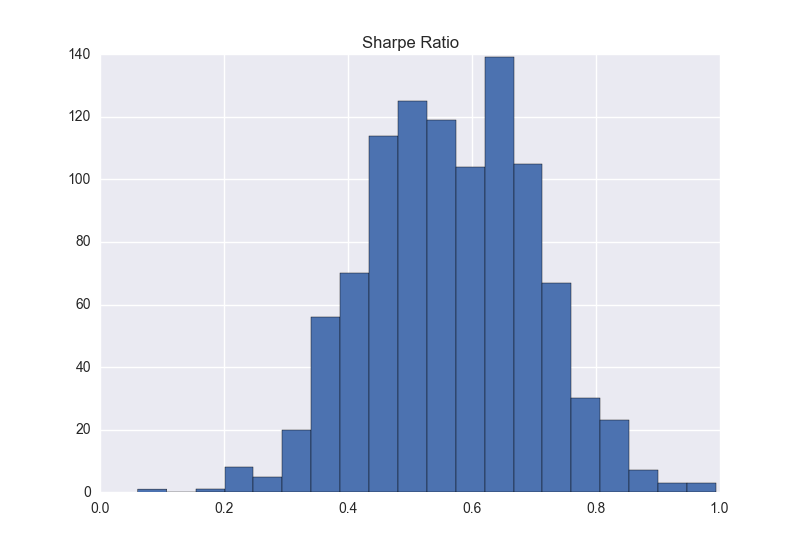# Low-level Interfaces¶

## Constructing Parameter Estimates¶

The bootstrap method apply can be use to directly compute parameter estimates from a function and the bootstrapped data.

This example makes use of monthly S&P 500 data.

import datetime as dt

import pandas as pd

start = dt.datetime(1951, 1, 1)
end = dt.datetime(2014, 1, 1)
sp500 = web.DataReader('^GSPC', 'yahoo', start=start, end=end)
low = sp500.index.min()
high = sp500.index.max()
monthly_dates = pd.date_range(low, high, freq='M')
monthly = sp500.reindex(monthly_dates, method='ffill')
returns = 100 * monthly['Adj Close'].pct_change().dropna()


The function will compute the Sharpe ratio – the (annualized) mean divided by the (annualized) standard deviation.

import numpy as np
def sharpe_ratio(x):
return np.array([12 * x.mean() / np.sqrt(12 * x.var())])


The bootstrapped Sharpe ratios can be directly computed using apply.

import seaborn
from arch.bootstrap import IIDBootstrap
bs = IIDBootstrap(returns)
sharpe_ratios = bs.apply(sr, 1000)
sharpe_ratios = pd.DataFrame(sharp_ratios, columns=['Sharpe Ratio'])
sharpe_ratios.hist(bins=20)## The Bootstrap Iterator¶

The lowest-level method to use a bootstrap is the iterator. This is used internally in all higher-level methods that estimate a function using multiple bootstrap replications. The iterator returns a two-element tuple where the first element contains all positional arguments (in the order input) passed when constructing the bootstrap instance, and the second contains the all keyword arguments passed when constructing the instance.

This example makes uses of simulated data to demonstrate how to use the bootstrap iterator.

import pandas as pd
import numpy as np

from arch.bootstrap import IIDBootstrap

x = np.random.randn(1000, 2)
y = pd.DataFrame(np.random.randn(1000, 3))
z = np.random.rand(1000, 10)
bs = IIDBootstrap(x, y=y, z=z)

for pos, kw in bs.bootstrap(1000):
xstar = pos  # pos is always a tuple, even when a singleton
ystar = kw['y']  # A dictionary
zstar = kw['z']  # A dictionary